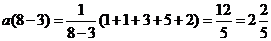## Delayed average

Delayed average or (in short dag ) is an average with delay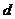is defined as arithmetic mean of the sequence up to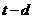,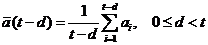(9)Diagram of delayed-average

The concept of delayed average is very useful when we are dealing with the sequence of measurement that grows over time. As the length of sequence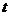is increasing by time, sometimes we would like to know the time average up to some time before the latest measurement. Delaymust be positive integer. When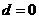, the delayed average is simply the average itself. If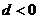, we are dealing with prediction, that we will address in later chapter. Of course,is not always represents the length of full sequence.is simply the total number of elements in our consideration that we want to take the average, counted from the beginning of the sequence.

Example

We have sequence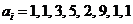. Let the delay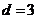, and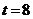, then